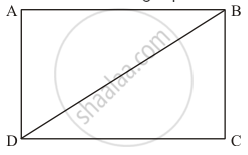Advertisement Remove all ads

# Diagonals Necessarily Bisect Opposite Angles in a - Mathematics

MCQ

Diagonals necessarily bisect opposite angles in a

#### Options

• rectangle

• parallelogram

• isosceles trapezium

• square

Advertisement Remove all ads

#### Solution

From the given choices, only in a square the diagonals bisect the opposite angles.

Let us prove it.

Take the following square ABCD with diagonal AD.In ΔABD and ΔCBD:

AD = BC (Opposite sides of a square are equal.)

BD = BD (Common)

AB = DC  (Opposite sides of a square are equal.)

Thus,

ΔABD ≅ ΔCBD (By SSS Congruence Rule)

By Corresponding parts of congruent triangles property we have:

∠ABD = ∠CBD

∠ADB = ∠CDB

Therefore, in a square the diagonals bisect the opposite angles.

Hence the correct choice is (d).

Is there an error in this question or solution?
Advertisement Remove all ads

#### APPEARS IN

RD Sharma Mathematics for Class 9
Chapter 13 Quadrilaterals
Q 5 | Page 71
Advertisement Remove all ads

#### Video TutorialsVIEW ALL 

Advertisement Remove all ads
Share
Notifications

View all notifications

Forgot password?
Course